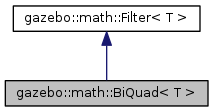##gazebo::math::BiQuad< T > Class Template Reference

`#include <math/gzmath.hh>`

Inheritance diagram for gazebo::math::BiQuad< T >:[legend]

## Public Member Functions

Constructor. More...

Constructor. More...

virtual const T & process (const T &_x)
Update the filter's output. More...

void SetFc (double _fc, double _fs)
Set the cutoff frequency and sample rate. More...

void SetFc (double _fc, double _fs, double _q)
Set the cutoff frequency, sample rate and Q coefficient. More...

virtual void SetValue (const T &_val)
Set the current filter's output. More...Public Member Functions inherited from gazebo::math::Filter< T >
virtual ~Filter ()
Destructor. More...

virtual const T & GetValue ()
Get the output of the filter. More...

## Protected Attributes

double a0
Input gain control coefficients. More...

double a1

double a2

double b0

double b1

double b2

x1
Gain of the feedback coefficients. More...

x2

y1

y2Protected Attributes inherited from gazebo::math::Filter< T >
y0
Output. More...

## Detailed Description

### template<class T> class gazebo::math::BiQuad< T >

http://www.earlevel.com/main/2003/03/02/the-bilinear-z-transform/

## Constructor & Destructor Documentation

template<class T>
inline

Constructor.

template<class T>
inline

Constructor.

Parameters
 [in] _fc Cutoff frequency. [in] _fs Sample rate.

## Member Function Documentation

template<class T>
 virtual const T& gazebo::math::BiQuad< T >::process ( const T & _x )
inlinevirtual

Update the filter's output.

Parameters
 [in] _x Input value.
Returns
The filter's current output.
template<class T>
 void gazebo::math::BiQuad< T >::SetFc ( double _fc, double _fs )
inlinevirtual

Set the cutoff frequency and sample rate.

Parameters
 [in] _fc Cutoff frequency. [in] _fs Sample rate.

Implements gazebo::math::Filter< T >.

template<class T>
 void gazebo::math::BiQuad< T >::SetFc ( double _fc, double _fs, double _q )
inline

Set the cutoff frequency, sample rate and Q coefficient.

Parameters
 [in] _fc Cutoff frequency. [in] _fs Sample rate. [in] _q Q coefficient.
template<class T>
 virtual void gazebo::math::BiQuad< T >::SetValue ( const T & _val )
inlinevirtual

Set the current filter's output.

Parameters
 [in] _val New filter's output.

Reimplemented from gazebo::math::Filter< T >.

## Member Data Documentation

template<class T>
protected

Input gain control coefficients.

template<class T>
protected
template<class T>
protected
template<class T>
protected
template<class T>
protected
template<class T>
protected
template<class T>
protected

Gain of the feedback coefficients.

template<class T>